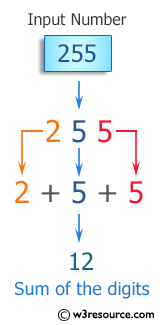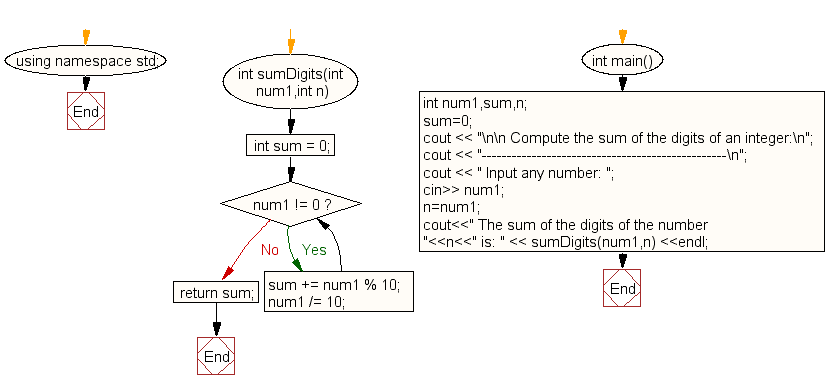﻿ C++ : Compute the sum of the digits of an integer

# C++ Exercises: Compute the sum of the digits of an integer using function

## C++ For Loop: Exercise-84 with Solution

Write a C++ program to compute the sum of the digits of an integer using a function.

Visual Presentation:Sample Solution:-

C++ Code :

``````#include <iostream> // Including the input-output stream library
using namespace std; // Using standard namespace

// Function to calculate the sum of the digits of a number
int sumDigits(int num1, int n)
{
int sum = 0; // Initializing sum to zero
while (num1 != 0) // Loop to sum the digits of the number
{
sum += num1 % 10; // Adding the last digit of 'num1' to 'sum'
num1 /= 10; // Removing the last digit from 'num1'
}
return sum; // Returning the total sum of the digits
}

int main() // Main function
{
int num1, sum, n; // Declaring three integer variables: num1, sum, n
sum = 0; // Initializing the variable 'sum' to zero

// Displaying a message to compute the sum of the digits of an integer
cout << "\n\n Compute the sum of the digits of an integer:\n";
cout << "-------------------------------------------------\n";

// Asking the user to input any number
cout << " Input any number: ";
cin >> num1; // Taking user input for 'num1'
n = num1; // Assigning the value of 'num1' to 'n' to preserve the original number

// Displaying the sum of the digits of the original number using the function
cout << " The sum of the digits of the number " << n << " is: " << sumDigits(num1, n) << endl;
}
``````

Sample Output:

```Compute the sum of the digits of an integer:
-------------------------------------------------
Input any number: 255
The sum of the digits of the number 255 is: 12
```

Flowchart:C++ Code Editor: